Next: 4.2 Lens Aberrations and Up: 4.1 Principles of Fourier Previous: 4.1.4 Köhler Illumination of

## 4.1.5 Vector-Valued Extension

The preceding derivation of the scalar theory of Fourier optics neglects the vector nature of light. For high numerical aperture systems, e.g., for NA = 0.5 and above, the approximations and simplifications made throughout fail and the oblique propagation of the waves becomes important. The most obvious vector diffraction effects arise from polarization in the illumination producing orientation-dependent asymmetries in the printed patterns. For example, in the case of phase-shifting masks or off-axis illumination the aerial image strongly depends on whether polarization parallel or perpendicular to the lines and spaces is used. In this section we follow the imaging part of Yeung's key work  and summarize the fundamental results therefrom using our notation.

The scalar amplitude U(x, y) used so far has to be replaced by vector functions E(x, y) and H(x, y) representing the electric and magnetic field, respectively. Assuming again a periodic mask, the two field vectors can be expanded into a plane wave superposition corresponding to the Fourier series of (4.51):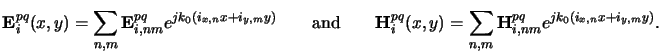(4.51)

The lateral components ix, n and iy, m of the wavevectors inm belonging to the image space follow from (4.47) to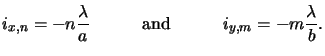(4.52)

Note that the magnification M is eliminated because inm is defined in the image space. The Fourier coefficients Ei, nmpq and Hi, nmpq in the above plane wave expansion are--similar to (4.52)--given by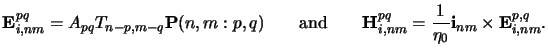(4.53)

The right equation ensures that the electric, magnetic, and orientation vector form a right-handed orthogonal triad. The left equation is equivalent to the scalar formula (4.52). Instead of the scalar pupil function P(n, m) we have now a vector-valued pupil function P(n, m : p, q) that also depends on the source point position (p, q) beside the diffraction order (n, m). Following  we write P(n, m : p, q) as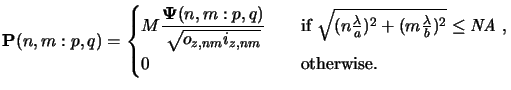(4.54)

Here,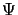(n, m : p, q) denotes the polarization vector. For further calculations it is convenient to decompose the polarization vector(n, m : p, q) into a transversal-electric (TE) and a transversal-magnetic (TM) mode. In case of TE-illumination the y-component of the electric field vanishes in the source space, for TM-illumination the magnetic field in the image space is transverse to the y-axis. The overall polarization state is composed like(4.55)

The two vectorsTE(n, m : p, q) andTM(n, m : p, q) are orthogonal and the two angles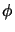and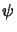determine the actual polarization state. The illumination is said to be linearly polarized in (cos, sin)-direction if= 0, circularly polarized for=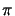/4 and=/2, and elliptically polarized otherwise. In case of unpolarized light the images for TE- and TM-modes have to be calculated separately with=/4 and incoherently superposed afterwards.

Expressions for the two fundamental modes are found by tracing the ray paths through the optical system as illustrated in Figure 4.6. For the sake of definiteness we first summarize the discretization of the wavevectors occurring in the three relevant spaces: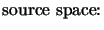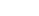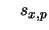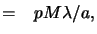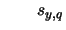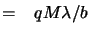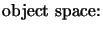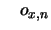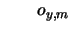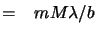(4.56)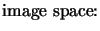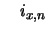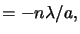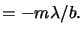As can be seen, the three vectors spq, onm, and inm lie in a common plane usually called meridional plane. Due to singularities the following two different cases have to be studied separately. The derivation of the provided formulae can be found in .
• Vertical incident ray or (n, m) = (0, 0). The TE- and TM-polarization vectors are given by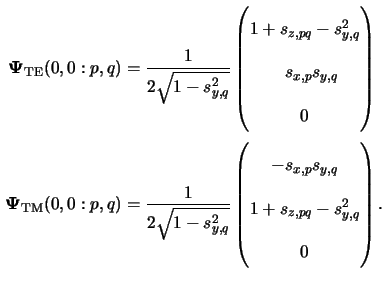• Oblique incident rays or (n, m)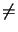(0, 0). Here, the TE- and TM-polarization vectors are further decomposed into a parallel and perpendicular vector to the meridional plane, i.e.,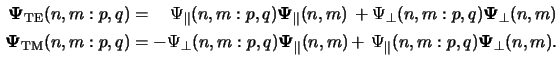The two amplitudes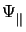(n, m : p, q) and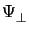(n, m : p, q) are given by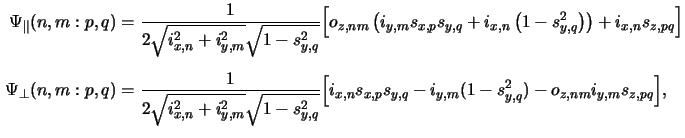and the two unit vectors|| (n, m) and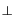(n, m) equal to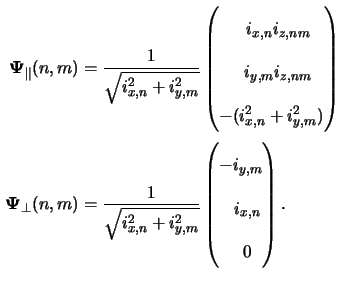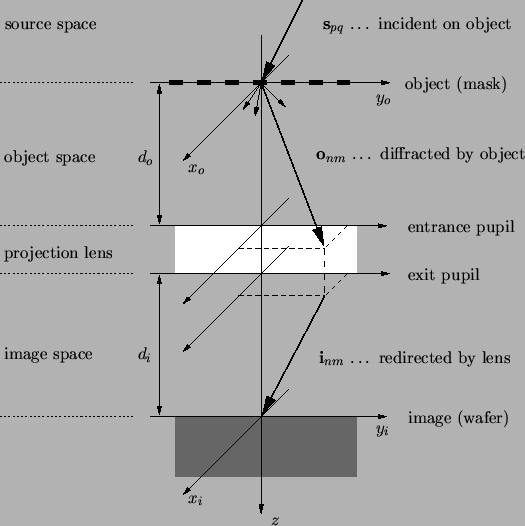The norm |P| of the vector-valued pupil functionm will be of interest for the energy normalization in case of more than one illuminating point source (cf. Section 4.3.1). It follows from (4.58) to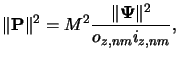(4.57)

whereby due to the orthogonality of the fundamental TE- and TM-modes the norm || of the polarization vector obeys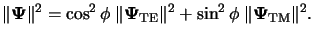(4.58)

It can be further shown that the following relation holds: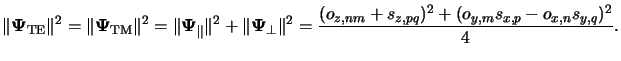(4.59)

Finally, the image intensity Iipq(x, y) due to one source point excitation (p, q) equals to the vertical component of the Poynting vector Sipq = Eipq x Hipq, i.e.,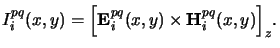(4.60)

#### Footnotes

... functionm
For the sake of a compact notation we suppress the dependence on the indices (n, m) and (p, q) for the moment.Next: 4.2 Lens Aberrations and Up: 4.1 Principles of Fourier Previous: 4.1.4 Köhler Illumination of
Heinrich Kirchauer, Institute for Microelectronics, TU Vienna
1998-04-17